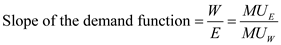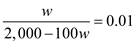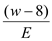# Quiz 10: Labor Unions

The slope of indifference curve and the value of the slope of workers' demand curve are taken in to the account while measuring the utility. The following is a measurement of the wage rate that would be demanded by a union.The given slope is 0.01. Inserting the labor demand function instead of E ,After solving, the above equation indicates that a monopoly union is likely to demand a wage rate of \$10. At the wage rate of \$10, the employers will hire 1,000 workers to maximize its output.
a) A Monopoly determines the number of workers to be employed by the point of tangency between the labor demand curve and the utility curve of the union. This implies, at the point of equilibrium, the slope of the labor demand curve and the slope of the indifference curve should be equal. The slope of the given labor demand curve is 0.01E. The slope of the utility function is the ratio of marginal utilities of wage increase and employment:Given the competitive wage rate w* = \$8 per hour, the slope of the utility function becomes. First equilibrate the two slopes and note that:Use the labor demand curve and the equation found before to compute the wage as demanded by the monopoly union:Hence the monopoly union would demand a wage rate of \$14. Under this union contract, as much as 600 workers will be employed. b) Previously, the union had demanded \$10 as a wage rate and at that wage rate, 1,000 workers were hired by the firm. The results are different because when the union has a utility that depends on the competitive wage rate, union observe that the demand curve is more inelastic and they can demand a high wage rate.